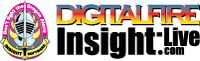•The secret to cool bodies and glazes is a lot of testing.
•The secret to know what to test is material and chemistry knowledge.
•The secret to learning from testing is documentation.
•The place to test, do the chemistry and document is an account at https://insight-live.com
•The place to get the knowledge is https://digitalfire.com

Sign-up at https://insight-live.com today.

Rice Husk Ash

 Oxide Analysis Formula CaO 0.49% 0.311 MgO 0.22% 0.194 K2O 0.91% 0.344 Na2O 0.26% 0.149 P2O5 0.01% 0.003 TiO2 0.16% 0.071 Al2O3 1.01% 0.352 SiO2 96.70% 57.223 Fe2O3 0.05% 0.011 MnO 0.19% 0.095 Oxide Weight 3556.47 Formula Weight 3556.47

The rice husk ash process produces an organic amorphous silica of very small particle size.

XML for Import into INSIGHT

<?xml version="1.0" encoding="UTF-8"?> <material name="Rice Husk Ash" descrip="" searchkey="" loi="0.00" casnumber=""> <oxides> <oxide symbol="CaO" name="Calcium Oxide, Calcia" status="" percent="0.490" tolerance=""/> <oxide symbol="MgO" name="Magnesium Oxide, Magnesia" status="" percent="0.220" tolerance=""/> <oxide symbol="K2O" name="Potassium Oxide" status="" percent="0.910" tolerance=""/> <oxide symbol="Na2O" name="Sodium Oxide, Soda" status="" percent="0.260" tolerance=""/> <oxide symbol="P2O5" name="Phosphorus Pentoxide" status="" percent="0.010" tolerance=""/> <oxide symbol="TiO2" name="Titanium Dioxide, Titania" status="" percent="0.160" tolerance=""/> <oxide symbol="Al2O3" name="Aluminum Oxide, Alumina" status="" percent="1.010" tolerance=""/> <oxide symbol="SiO2" name="Silicon Dioxide, Silica" status="" percent="96.700" tolerance=""/> <oxide symbol="Fe2O3" name="Iron Oxide, Ferric Oxide" status="" percent="0.050" tolerance=""/> <oxide symbol="MnO" name="Manganous Oxide" status="" percent="0.190" tolerance=""/> </oxides> </material>